﻿ Built-in Functions
QPR Knowledge Base 2017.1

Built-in Functions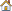Metrics User's Guide > Using QPR Metrics > Formulas:

Built-in FunctionsBuilt-in Functions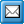Overview of the Built-in Functions

There are many built-in functions available to help you define your formulas. The most commonly used of these functions are as follows:

To take the average of values: AVERAGE(X1, X2,...)

To take the summation of values: SUM(X1, X2,...)

To take the minimum of values: MIN(X1, X2,...)

To take the maximum of values: MAX(X1, X2,...)

To take the product of values: PROD(X1, X2,...)

When you call a function, you must supply an actual values for all function's arguments, and separate them with a comma (','). Formula functions are not case sensitive and therefore the formula functions can be entered in both uppercase and lowercase letters.

Functions can also contain constants unless otherwise specified. For more information on constant types, see Constants.

When function parameters are derived from other functions or expressions, it is possible that parameters will not get values at all. Since regular functions cannot handle these so-called NIL values, the calculation will end to an error. In order to ignore NIL values in your formula calculations, you can enter the underscore character "_" after the function name. Therefore AVERAGE_(X1, X2,...), SUM_(X1, X2,...), MIN_(X1, X2,...), etc., are all valid functions with the exception of ignoring all supplied NIL values.

Functions that return lists of values (such as CUMULATIVE and RANGE) should not be used in formulas directly but in combination with aggregate functions such as SUM, AVERAGE, MAX, or MIN.

For example, the formula AVERAGE_(NIL,2,NIL,3), where NIL represents an undefined (null) value, will be calculated as AVERAGE(2,3).

Function Categories

In addition, there are different operators and wildcards available:

Consolidation Functions

 ACCUMULATE Returns list of values, accumulated to the period level of the element in which the function is. CUMULATIVE Returns a list of cumulative values, one per each expression. SUM Returns the sum of the arguments.

ACCUMULATE

Description

Returns list of values, accumulated to the period level of the element in which the function is.

Syntax

ACCUMULATE(expression_list, accumulation_rule)

Parameters: expression_list, accumulation_rule

expression_list contains an even number of expressions separated by commas. The list has references to value series first and then either a date series or NIL reference for each value series.

accumulation_rule is one of the following values enclosed in double quotes: DEFAULT, SUM, SUM_, AVERAGE, AVERAGE_, MIN, MAX, FIRST, LAST, or LATEST.

Examples

ACCUMULATE(mea1.act(), NIL, "default") : Accumulate mea1.act() using accumulation rule of mea1 (same as "mea1.act()")

ACCUMULATE(mea1.act(), NIL, "sum_") : Accumulate mea1.act() using accumulation rule sum_ (override element's own accumulation rule with sum_)

ACCUMULATE(mea1.act(), mea1.date(), "average_") : Accumulate mea1.act() using accumulation rule average_. Accumulated values are selected (targeted to periods)

according to dates found in mea1.date().

ACCUMULATE(mea1.act(), mea2.act(), NIL, mea3.dates(), "default") : accumulate values from mea1.act() and mea2.act() with the element's own accumulation rule.

Select accumulated values for mea2.act() on the basis of the dates found in mea3.dates() series.

CUMULATIVE

Description

Returns a list of cumulative values, one per each expression. In the case there are null values in the results, they must be controlled with container functions such as SUM_ or AVERAGE_ (for example, SUM_(CUMULATIVE(?.act(), 1, SUM_)) or the CUMULATIVE_ function must be used.

Syntax

CUMULATIVE(expression_list,relative level,formula )

CUMULATIVE_(expression_list,relative level,formula )

Parameter: expression_list

A list of expressions separated by commas. An expression is a reference to element's series on a certain period.

Parameter: relative level

An integer value stating which period level will be used in the calculation. The number is a relative value, pointing upward in the period level hierarchy. Values less than 1 are considered invalid.

Parameter: formula

This parameter determines which function will be used for calculating the cumulative values. The following function names are valid: SUM, SUM_, AVERAGE, and AVERAGE_.

Examples

This function returns the average of all mea1 measure values. The calculation will start at the beginning of a period which is one level higher than the period level of sc1.mea1.

CUMULATIVE(sc1.mea1.act(),1,AVERAGE)

This function returns a cumulative sum for actual and alarm series of the current measure, scorecard and period. The calculation will start at the beginning of a period which is two levels higher than the period level of sc1.mea1.

CUMULATIVE(sc1.mea1.act(),sc1.mea1.alarm(),2,SUM)

This function calculates a cumulative sum of sales. Calculation is done with all scorecards' sales elements, closed series. Sum is cumulated one period level upwards from the sales-element's own period level. For instance, if sales is measured in day level and 'month' is next period level upwards from 'day',  then cumulative sum is calculated for monthly level.

SUM_(CUMULATIVE(?.SALES.CLOSED(), 1, SUM_))

Expressions

SUM

Description

Returns the sum of the arguments.

Syntax

SUM(X1, X2, ... )

SUM_(X1, X2, ... )

Parameter: X

Any numeric expression.

Examples

This function returns 16.

SUM(1,12,3)

Conversion and Rounding Functions

 CEIL Rounds a number up to the nearest integer value. FETCH Recalculates the series that is given as a parameter. FLOOR Rounds a number down to the nearest integer value. RANGE Returns a list of range values, one per each expression. ROUND Rounds the given number to an integer value. SIGN Returns an integer value depending on the parameters sign. TRUNC Truncates decimal number to integer number.

CEIL

Description

Rounds a number up to the nearest integer value.

Syntax

CEIL(number )

Parameter: number

The value to round.

Remarks

Both negative and positive values are always rounded up, i.e. negative values go to the next integer value towards zero, positive values go to the next integer value away from zero. Measure identifiers can be used instead of numbers.

Examples

This function returns 2:

CEIL(1.23459)

This function returns 149:

CEIL(148.24)

This function returns -1:

CEIL(-1.5)

FETCH

Description

Recalculates the value of the given series by using the period for which the calculation is done, instead of using the value of the matching period in the period level of the series given. If the input type of the series is not formula, returns the values of the parameter series.

Syntax

FETCH(identifier )

Parameter: identifier

The identifier of the series for which the value is to be recalculated.

FLOOR

Description

Rounds a number down to the nearest integer value.

Syntax

FLOOR(number )

Parameter: number (or measure identifier )

The value to round.

Remarks

Both negative and positive values are rounded down.

Examples

This function returns 1:

FLOOR(1.23459)

This function returns -149:

FLOOR(-148.24)

RANGE

Description

Returns a list of range values, one per each expression. In the case there are null values in the results, they must be controlled with container functions such as SUM_ or AVERAGE_ (for example, SUM_(RANGE(?.ACT())).

Note: You can use also the abbreviated form R as this function's name.

Ignore null version of this function is not supported. Both ignore null values and normal versions of this function work similarly.

Syntax

RANGE(EXPRESSION1, EXPRESSION2, ... )

RANGE_(EXPRESSION1, EXPRESSION2, ... )

Parameter: EXPRESSION

Any valid expression.

Examples

This function returns the range value of mea1:

RANGE(mea1.act1(latest))

This function returns all range values of the latest period of all existing measures, where measure identifier is mea1 and series identifier is act1. Values will be separated by comma.

RANGE(?.mea1.act1(latest))

ROUND

Description

Rounds the given number to an integer value. Negative values are rounded symmetrically, i.e. -0.5 is rounded to -1.

Syntax

ROUND(number,amount of decimals)

Parameters:

Number, any value or measure identifier.

Amount of decimals, the precision to which the number is rounded, i.e. the number of decimals present in the result. Giving 0 rounds to the nearest even number. Note that the amount of decimals can also be negative.

Examples

This function returns 12.4:

ROUND(12.44, 1)

This function returns 12:

ROUND(12.44,0)

This function returns 10:

ROUND(12.44,-1)

This function returns 100:

ROUND(113,-2)

SIGN

Description

Returns an integer value depending on the parameters sign.

Syntax

SIGN(number )

Sign of X; =1 for X>0, =0 for X=0, =-1 for X<0

Examples

This function returns 1 :

SIGN(5)

This function returns 0 :

SIGN(0)

This function returns -1 :

SIGN(-5)

TRUNC

Alias

INT

Description

Truncates decimal number to integer number.

Syntax

TRUNC(number )

Parameter: number

A real number.

Examples

This function returns 1.

TRUNC(1.5134)

This function returns -13.

TRUNC(-13.15)

Date and Time Functions

 DATE Returns the current date. DATETIME Returns a number representing a datetime given as the parameter. DAY Returns the number of the day, of date. This is the number of days passed in the month. ENCODEDATE Returns a TDateTime type for a specified Year, Month, and Day. HOUR Returns the number of hours in a datetime. MINUTE Returns the number of minutes (0-59) in a datetime. MONTH Returns the month date is in. January is one. PERIODEND Returns period's last date PERIODSTART Returns period's first date. SECOND Returns the number of seconds (0-59) in a datetime. TIME Returns a number representing the Hour, Minute, and Second given as parameters. WEEKDAY Returns the day of the week of the specified date as an integer between 1 and 7. YEAR Returns the year date is in.

DATE

Description

The Date function returns the current date.

Syntax

DATE()

DATETIME

Description

The DateTime function returns a number representing a datetime given as the parameter. The value is a number representing time that has passed since 12/30/1899. The Year must be between 1 and 9999. Valid Month values are 1 through 12. Valid Day values are 1 through 28, 29, 30, or 31, depending on the Month value. For example, the possible Day values for month 2 (February) are 1 through 28 or 1 through 29, depending on whether or not the Year value specifies a leap year. Valid Hour values are 0 through 23. Valid Minute values are 0 through 59. Valid Second values are 0 through 59.

Syntax

DATETIME(Y,M,D,H,M,S)

DAY

Description

Returns the number of the day, of date. This is the number of days passed in the month.

Syntax

DAY(D)

The date specified in D.

DAYOFWEEK

Description

The DAYOFWEEK function returns the day of the week of the specified date as an integer between 1 and 7. Sunday is the first day of the week and Saturday is the seventh. Note that this function is included for backward compatibility only. Please use WEEKDAY instead.

Syntax

DAYOFWEEK(D)

The date specified in D.

ENCODEDATE

Description

EncodeDate returns a TDateTime type for a specified Year, Month, and Day. The TDateTime value is the number of days that have passed since 12/30/1899.

The Year must be between 1 and 9999. Valid Month values are 1 through 12. Valid Day values are 1 through 28, 29, 30, or 31, depending on the Month value. For example, the possible Day values for month 2 (February) are 1 through 28 or 1 through 29, depending on whether or not the Year value specifies a leap year.

Syntax

ENCODEDATE(Y,M,D)

The year is specified in Y, the month is specified in M and the date specified in D.

Example

This function returns 37,378.00 :

ENCODEDATE(2002,5,2)

EXTRACTDAY

Description

Returns the number of the day, of date. This is the number of days passed in the month. Note that this function is included for backward compatibility only. Please use DAY instead.

Syntax

EXTRACTDAY(D)

The date specified in D.

EXTRACTMONTH

Description

Returns the month date is in. January is one. Note that this function is included for backward compatibility only. Please use MONTH instead.

Syntax

EXTRACTMONTH(D)

The date specified in D.

EXTRACTYEAR

Description

Returns the year date is in. Note that this function is included for backward compatibility only. Please use YEAR instead.

Syntax

EXTRACTYEAR(D)

The date specified in D.

HOUR

Description

Returns the number of hours in a datetime.

Syntax

HOUR(D)

The datetime specified in D.

MINUTE

Description

Returns the number of minutes (0-59) in a datetime.

Syntax

MINUTE(D)

The datetime specified in D.

MONTH

Description

Returns the month date is in. January is one.

Syntax

MONTH(D)

The date specified in D.

PERIODEND

Description

Returns period's last date.

Syntax

PERIODEND()

PERIODSTART

Description

Returns period's first date.

Syntax

PERIODSTART()

SECOND

Description

Returns the number of seconds (0-59) in a datetime.

Syntax

Second(D)

The datetime specified in D.

TIME

Description

Returns a number representing the Hour, Minute, and Second given as parameters. Hour values are 0 through 23. Minute and Second values are 0 through 59

Syntax

TIME(H,M,S)

The time specified in H, M, and S.

WEEKDAY

Description

The WEEKDAY function returns the day of the week of the specified date as an integer between 1 and 7. Sunday is the first day of the week and Saturday is the seventh.

Syntax

WEEKDAY(D)

The date specified in D.

YEAR

Description

Returns the year date is in.

Syntax

YEAR(D)

The date specified in D.

Logical Functions

 IIF Tests the condition and returns the specified value. ISNULL Tests if the value of the parameter given to it is NULL. COALESCE Takes an element value or built-in formula function as an input. If the result is NIL (there is no value) the return value is given. Otherwise the input value is returned.

IIF

Description

Tests the condition and returns the specified value.

Syntax

IIF(condition , true_value, false_value )

Parameter: condition

Any logical expression. As evaluation order may affect the results, it is recommended to use parentheses with longer expressions, for example IIF(MEA1.ACT()+1=2, true, false) should be input as IIF((MEA1.ACT()+1)=2, true, false)

Parameter: true_value

The value to be returned if condition evaluates to True (i.e. <>0).

Parameter: false_value

The value to be returned if condition evaluates to False (i.e. =0).

Examples

This function returns 5 if the "Actual" value (series identifier "ACT") of MEA1 is greater than 10 and 2 if the "Actual" value of MEA1 is less than 10:

IIF(MEA1.ACT()>10, 5, 2)

ISNULL

Description

Tests if the value of the parameter given to it is NULL and returns 0 if the value is not NULL or 1 if it is NULL. If the parameter contains several measure values or it is a number list, the function returns a number list indicating the NULL value of each measure value or number in the list. Number lists are tested in listed order, but the testing order of measure values is not defined. Note: Using a function that ignores NULL values as the parameter for ISNULL is not supported. For example, functions like ISNULL(SUM_(NULL,1)) will return 1, even though it would seem they shouldn't.

Syntax

ISNULL(X)

Parameter: X can be any number, number list  or measure identifier.

Examples

When ?.ACT contains three measures with no NULL values:

This function returns [0 0 0]:

ISNULL(?.ACT)

When ?.ACT contains three measures with one having a NULL value:

This function returns [0 1 0]

ISNULL(?.ACT)

COALESCE

Description

The Coalesce function takes an element value or built-in formula function as an input. If the result is NIL (there is no value) the return value is given. Otherwise the input value is returned.

Syntax

COALESCE(Input Value, Return Value )

Input value can be either element's value for certain series or one of QPR Metrics's built-in functions.

Return value can be a number, element's value for a certain series or one of QPR Metrics's built-in functions.

Examples

In the following examples, the current period is period2 and Actual ("ACT") and Simulation ("SIM") are series of the elements. The elements MEA1 and MEA2 have the following values:

 For MEA1: For MEA2: Period 1, Actual value = 1 Period 2, Actual value = N/A Period 1, Actual value = 2 Period 1, Simulation value = N/A Period 2, Actual value = 2 Period 2, Simulation value = 3

This function, with Input value MEA1.ACT() and Return value 0, returns the value 0  because the input value is not available:

Coalesce(MEA1.ACT(), 0)

= Coalesce(N/A,0)

This function, with Input value SUM(MEA1.ACT(-1), MEA2.SIM()) and Return value MEA2.ACT(-1), returns the value 4 :

Coalesce(SUM(MEA1.ACT(-1), MEA2.SIM()), MEA2.ACT(-1))

= Coalesce(SUM(1,3),2)

= Coalesce(4,2)

Math and Trigonometry Functions

 ABS Returns the absolute value of the number. ACOS Returns the arc cosine. ARG Returns argument (phase) of X and Y. ASIN Returns the arc sine. ATAN Returns the arc tangent. COS Returns the cosine of an angle. COSH Returns the hyperbolic cosine. COTAN Returns the cotangent. EXP Returns e raised to a power number , where e is the base of the natural logarithms. FRAC Returns the fractional part of an argument. IPOWER Returns X raised to a power of Y. LG Returns the log base 10 for X. LN Returns the natural log of a real expression X. LOG Returns the log base 2 for X. POWER Raises Base to the power specified by Exponent. PROD Returns the product of arguments. RADIUS Returns the length of a circle's radius SIN Returns the sine of an angle. SINH Returns the hyperbolic sine. SQR Returns the square of a number. SQRT Returns the square root of a number. TAN Returns the tangent of an angle. TANH Returns the hyperbolic tangent. ZERO Returns 0 if the given argument is 0. Otherwise the function returns 1.

ABS

Description

Returns the absolute value of the number.

Syntax

ABS(number )

Parameter: number

the number argument can be any valid numeric expression.

Examples

These functions return 8 :

ABS(-8) and ABS(8)

ACOS

Description

Returns the arc cosine. Since arc cosine is the inverse function of cosine, it returns an angular radian value.

Syntax

ACOS(X)

Parameter: X, where -1 <= X <= 1

Examples

This function returns 1.0471975511965976 :

ACOS(0.5)

ASIN, ATAN, COS, COSH, COTAN, SIN, SINH, TAN, TANH.

ARG

Description

Returns argument (phase) of X and Y.

Syntax

ARG(X,Y)

Parameters: X and Y

the arguments X and Y can be a positive or negative decimal number.

Examples

This function returns 0.46 :

ARG(2,1)

This function returns 0 :

ARG(3,0)

ABS

ASIN

Description

Returns the arc sine. Since arc sine is the inverse function of sine, it returns an angular value.

Syntax

ASIN(X)

Parameter: X, where -1 <= X <= 1

Examples

This function returns 1.5707963267948966 :

ASIN(1)

ACOS, ATAN, COS, COSH, COTAN, SIN, SINH, TAN, TANH.

ATAN

Description

Returns the arc tangent. Since arc tangent is the inverse function of tangent, it returns an angular radian value.

Syntax

ATAN(X)

Parameter: X, where X can be any number.

Examples

This function returns 0.7853981633974483 :

ATAN(1)

ASIN, ACOS, COS, COSH, COTAN, SIN, SINH, TAN, TANH.

COS

Description

Returns the cosine of an angle.

Syntax

COS(angle )

Parameter: angle

Angle is any valid numeric expression, measured in radians.

Examples

This function returns -0.9899924966004454 :

COS(3)

ASIN, ACOS, ATAN, COSH, COTAN, SIN, SINH, TAN, TANH.

COSH

Description

Returns the hyperbolic cosine.

Syntax

COSH(angle )

Parameter: angle

Angle is any valid numeric expression, measured in radians.

Examples

This function returns 10.067661995777765 :

COSH(3)

ASIN, ACOS, ATAN, COS, COTAN, SIN, SINH, TAN, TANH.

COTAN

Description

Returns the cotangent.

Syntax

COTAN(angle )

Parameter: angle

Angle is any valid numeric expression, measured in radians.

Examples

This function returns 1.461696 :

COTAN(0.6)

ASIN, ACOS, ATAN, COS, COSH, SIN, SINH, TAN, TANH.

EXP

Description

Returns e raised to a power number , where e is the base of the natural logarithms.

Syntax

EXP(number ) =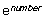Parameter: number

the number argument can be any valid numeric expression.

Examples

This function returns 1.

EXP(0)

This function returns 2.718282 :

EXP(1)

LOG

FRAC

Description

Returns the fractional part of an argument.

Syntax

FRAC(number )

Parameter: the number argument can be any valid numeric expression.

Examples

This function returns 0.1415 :

FRAC 3.1415

IPOWER

Description

Returns X raised to a power of Y. This function converts the arguments into integer values (by ignoring the decimal part) before executing the calculation.

Syntax

IPOWER(X,Y)

Parameters: X can be any valid numeric expression. Y is an integer value.

Examples

This function returns 8:

IPOWER(2,3)

This function returns 27 (the decimal part is ignored):

IPOWER(3.2,3)

LG

Description

The LG function returns the log base 10 for X.

Syntax

LG(X)

Parameter: X > 0.

Examples

10^X = 1000, to find out the X use LG function.

X= lg(1000) = 3

6^X = 1296, to find out the X use LG function

X= lg(1296)/lg(6) = 4

LN

Description

LN returns the natural log of a real expression X.

Syntax

LN(X)

Parameter: X > 0.

Examples

This function returns 0.693147 :

LN(2)

This function returns 2.302585 :

LN(10)

LG, LN

LOG

Description

The LOG function returns the log base 2 for X.

Syntax

LOG(X)

Parameter: X > 0.

Examples

2^X= 256, to find out the X, use LOG function.

X = LOG(256) = 8

LG, LN

POWER

Description

Power raises Base to the power specified by Exponent.

Syntax

POWER(X,Y)

Parameter:

X, base can be any valid numeric expression.

Y, exponent is a floating point value.

Examples

This function returns 4 :

POWER(16,0.5)

PROD

Description

Product of arguments.

Syntax

PROD(X1,X2,...)

PROD_(X1,X2,...)

Parameter:X ,

Any valid numeric expression.

Description

Returns the length of a circle's radius, where the radius is the hypotenuse of the right-angled triangle with vertices at (0,0), (X,Y), and (X,0).

Syntax

SQRT

SIN

Description

Returns the sine of an angle.

Syntax

SIN(angle )

Parameter: angle

Angle is any valid numeric expression, measured in radians.

Examples

This function returns 0.1411200080598672.

SIN(3)

ASIN, ACOS, ATAN, COS, COSH, COTAN, SINH, TAN, TANH.

SINH

Description

Returns the hyperbolic sine.

Syntax

SINH(angle )

Parameter: angle

Angle is any valid numeric expression, measured in radians.

Examples

This function returns 10.017874927409903.

SINH(3)

ASIN, ACOS, ATAN, COS, COSH, COTAN, SIN, TAN, TANH.

SQR

Description

Returns the square of a number.

Syntax

SQR(number )

Parameter: number

Any valid numeric expression greater than or equal to 0.

Examples

This function returns 9.

SQR(3)

SQRT

Description

Returns the square root of a number.

Syntax

SQRT(number )

Parameter: number

A non-negative integer or a decimal number.

Examples

This function returns 16.

SQRT(256)

TAN

Description

Returns the tangent of an angle.

Syntax

TAN(angle )

Parameter: angle

Any valid numeric expression, measured in radians.

Examples

This function returns -0.1425465430742778.

TAN(3)

ASIN, ACOS, ATAN, COS, COSH, COTAN, SIN, TANH.

TANH

Description

Returns the hyperbolic tangent.

Syntax

TANH(angle )

Parameter: angle

Any valid numeric expression, measured in radians.

Examples

This function returns 0.9950547536867306.

TANH(3)

ASIN, ACOS, ATAN, COS, COSH, COTAN, SIN, TAN.

ZERO

Description

Returns 0 if the given argument is 0. Otherwise the function returns 1.

Syntax

ZERO(number ), =0 for X=0, =1 for X<>0

Parameter: number

Can be any valid numeric expression.

Examples

This function returns 0:

ZERO(0)

This function returns 1:

ZERO(135)

Statistical Functions

 AVERAGE Returns the average of the supplied numbers. The result of AVERAGE is also known as the arithmetic mean. COUNT Returns the length of the given number list, object vector or string list. MA Returns the moving average based on the number of periods given with the delay given. MAX Returns the largest value in the specified list of numbers. MEDIAN Returns the median in the specified list of numbers. MIN Returns the smallest value in the specified list of numbers. RANDOM Returns a random value between 0 and 1. STDEV Returns the standard deviation based on a sample. STDEVP Returns the standard deviation based on the entire population given as arguments. VAR Returns variance based on a sample. VARP Returns variance based on the entire population.

AVERAGE

Description

Returns the average of the supplied numbers. The result of AVERAGE is also known as the arithmetic mean. Numbers can be replaced by measure identifiers.

Syntax

AVERAGE(number_list )

AVERAGE_(number_list )

Parameter: number_list

A list of numbers or measure identifiers separated by commas. All numeric values (including 0) can be used.

Examples

This function returns 8.25:

AVERAGE(5, 6, 8, 14)

CEIL, FLOOR, MAX, MIN, ROUND.

COUNT

Description

Returns the length of the given number list, object vector or string list. If the parameter given to it is NULL, 0 is returned. If no parameters are given, 1 is returned.

Syntax

COUNT(list, x )

Parameters: list, x

List is any number list, object vector or string list.

X is either 0 or 1. Defines whether NULL values are included in the count or not. 0 = NULL values are not included (default), 1 = NULL values are included.

Examples

When ?.ACT has the following values: [0 NULL 1].

This function returns NULL:

COUNT(?.ACT)

This function returns 2:

COUNT_(?.ACT)

To calculate the total number of child measures having ACT-series (no matter if there are NULL values or not), the following function can be used:

COUNT_(?.ACT, 1)

MA

Description

Returns the moving average based on the number of periods given with the delay given.

Syntax

MA(dataseries, X, Y)

Parameters:

dataseries is the dataseries used for moving average calculation.

X is the number of periods used for the average calculation.

Y is the number of periods used as delay in the calculation

Examples

When MEA123.ACT() has the values [1,0 2,0 3,0 4,0 5,0 6,0 7,0 8,0 9,0 10,0]:

MA(MEA123.ACT(),3,0) returns [null null 2,0 3,0 4,0 5,0 6,0 7,0 8,0 9,0]

MA(MEA123.ACT(),3,2) returns [null null null null 2,0 3,0 4,0 5,0 6,0 7,0]

MAX

Description

Returns the largest value in the specified list of numbers.

Syntax

MAX(number_list )

MAX_(number_list )

Parameter: number_list, where number_list is a list of numbers separated by commas.

When the list contains one or more blank values, then the value returned is blank.

Examples

This function returns 500:

MAX(50, 100, 150, 500, 200)

MEDIAN

Description

Returns the median in the specified list of numbers.

Syntax

MEDIAN(number_list)

MEDIAN_(number_list)

Parameter: number_list, where number_list is a list of numbers separated by commas.

The number_list can also be a dataseries.

Examples

When MEA123.ACT() has the values [1 2 3 4 5 6 7 8 9 10]:

MEDIAN(MEA123.ACT(AllToDate)) returns [1,0 1,5 2,0 2,5 3,0 3,5 4,0 4,5 5,0 5,5]

MEDIAN(MEA123.ACT(All)) returns [5,5 5,5 5,5 5,5 5,5 5,5 5,5 5,5 5,5 5,5]

MIN

Description

Returns the smallest value in the specified list of numbers.

Syntax

MIN(number_list )

_MIN(number_list )

Parameter: number_list, where number_list is a list of numbers separated by commas.

When the list contains one or more blank values, then the value returned is blank.

Examples

This function returns 50:

MIN(50, 100, 150, 500, 200)

RANDOM

Description

Returns a random value between 0 and 1.

Syntax

RANDOM()

The Random function returns a random number within the range 0 <= X < 1.

STDEV

Description

Returns the standard deviation based on a sample. STDEV assumes that its arguments are a sample of the population. If your data represents the entire population, compute the standard deviation using STDEVP. The standard deviation is calculated using the "nonbiased" or "n-1" method using the following formula: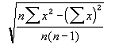Syntax

STDEV(number_list)

STDEV_(number_list)

Parameter: number_list

A list of numbers or dataseries separated by commas.

Examples

When MEA123.ACT() has the values [1,0 2,0 3,0 4,0 5,0 6,0 7,0 8,0 9,0 10,0]:

STDEV(MEA123.ACT(AllToDate)) returns [null 0,7 1,0 1,3 1,6 1,9 2,2 2,4 2,7 3,0]

STDEV(MEA123.ACT(All)) returns [3,0 3,0 3,0 3,0 3,0 3,0 3,0 3,0 3,0 3,0]

STDEVP

STDEVP

Description

Returns the standard deviation based on the entire population given as arguments. STDEVP assumes that its arguments are the entire population. If your data represents a sample of the population, compute the standard deviation using STDEV. For large sample sizes, STDEV and STDEVP return approximately equal values. The standard deviation is calculated using the "biased" or "n" method using the following formula: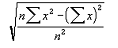Syntax

STDEVP(number_list)

STDEVP_(number_list)

Parameter: number_list

A list of numbers or dataseries separated by commas.

Examples

When MEA123.ACT() has the values [1,0 2,0 3,0 4,0 5,0 6,0 7,0 8,0 9,0 10,0]:

STDEVP(MEA123.ACT(AllToDate)) returns [0,0 0,5 0,8 1,1 1,4 1,7 2,0 2,3 2,6 2,9]

STDEVP(MEA123.ACT(All)) returns [2,9 2,9 2,9 2,9 2,9 2,9 2,9 2,9 2,9 2,9]

STDEV

VAR

Description

Returns variance based on a sample. VAR assumes that its arguments are a sample of the population. If your data represents the entire population, then compute the variance using VARP. VAR is calculated using the following formula: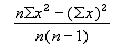Syntax

VAR(number_list)

VAR_(number_list)

Parameter: number_list

A list of numbers or dataseries separated by commas.

Examples

When MEA123.ACT() has the values [1,0 2,0 3,0 4,0 5,0 6,0 7,0 8,0 9,0 10,0]:

VAR(MEA123.ACT(AllToDate)) returns [null 0,5 1,0 1,7 2,5 3,5 4,7 6,0 7,5 9,2]

VAR(MEA123.ACT(All)) returns [9,2 9,2 9,2 9,2 9,2 9,2 9,2 9,2 9,2 9,2]

VARP

VARP

Description

Returns variance based on the entire population. VARP assumes that its arguments are the entire population. If your data represents a sample of the population, then compute the variance using VAR. VARP is calculated using the following formula: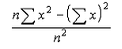Syntax

VARP(number_list)

VARP_(number_list)

Parameter: number_list

A list of numbers or dataseries separated by commas.

Examples

When MEA123.ACT() has the values [1,0 2,0 3,0 4,0 5,0 6,0 7,0 8,0 9,0 10,0]:

VARP(MEA123.ACT(AllToDate)) returns [0,0 0,3 0,7 1,3 2,0 2,9 4,0 5,3 6,7 8,3]

VARP(MEA123.ACT(All)) returns [8,3 8,3 8,3 8,3 8,3 8,3 8,3 8,3 8,3 8,3]

Text Processing Functions

 MID Returns a substring of S. The substring is l characters long, starting from s. LEFT Returns l leftmost characters of S. RIGHT Returns l rightmost characters of S. LENGTH Returns the number of characters in S. LOWER Returns S converted to lower case. UPPER Returns S converted to upper case. REPLACE Replaces all occurrences of o in S by n, after which the string is returned. CONCATENATE Returns a string which is formed by concatenating strings given as parameters. SMAX Returns the largest of the arguments. NULL is considered to be the smallest possible string. SMIN Returns the smallest of the arguments. NULL is considered to be the smallest possible string. FIND Returns the index of the first occurrence of s in S starting from 1. If s doesn't exist in S, 0 is returned. TONUMBER Returns the best possible numeric interpretation of S, or 0 if S cannot be interpreted as a number at all. TRIM Returns the text given to it from which any leading and/or tailing whitespaces are removed. REVERSE Returns the text given to it in reverse order. REPEAT Returns the text given to it repeated n times.

Note that all the functions listed in this section take string(s) as parameter(s). If a number is given to them as a parameter when string is expected, the number is converted to string.

MID

Description

Returns a substring of S. The substring is l characters long, starting from s.

Syntax

MID(S,s,l)

Parameter: S, String, the text to process

Parameter: s, Integer, index of the start position (starting from 1)

Parameter: l, Integer, number of characters to include

Examples

This function returns "xam":

MID("Example",2,3)

LEFT

Description

Returns l leftmost characters of S.

Syntax

LEFT(S,l)

Parameter: S, String, the text to process

Parameter: l, Integer, number of characters to include

Examples

This function returns "Exa":

LEFT("Example",3)

RIGHT

Description

Returns l rightmost characters of S.

Syntax

RIGHT(S,l)

Parameter: S, String, the text to process

Parameter: l, Integer, number of characters to include

Examples

This function returns "ple":

RIGHT("Example",3)

LENGTH

Description

Returns the number of characters in S.

Syntax

LENGTH(S)

Parameter: S, String, the text to process

Examples

This function returns 7:

LENGTH("Example")

LOWER

Description

Returns S converted to lower case.

Syntax

LOWER(S)

Parameter: S, String, the text to process

Examples

This function returns "example":

LOWER("EXAmple")

UPPER

Description

Returns S converted to upper case.

Syntax

UPPER(S)

Parameter: S, String, the text to process

Examples

This function returns "EXAMPLE":

UPPER("EXAmple")

REPLACE

Description

Replaces all occurrences of o in S by n, after which the string is returned.

Syntax

REPLACE(S,o,n)

Parameter: S, String, the text to process

Parameter: o, String, the text to search for

Parameter: n, String, the text to replace the searched string

Examples

This function returns "FooForFoz":

REPLACE("FooBarBaz","Ba","Fo")

CONCATENATE

Description

Returns a string which is formed by concatenating strings given as parameters.

Syntax

CONCATENATE(S1...n)

Parameter: S1...n, Any number of strings to be concatenated

Examples

This function returns "Example":

CONCATENATE("Ex","amp","le")

SMAX

Description

Returns the largest of the arguments. NULL is considered to be the smallest possible string.

Syntax

SMAX(S1...n)

Parameter: S1...n, Any number of strings to be compared

Examples

This function returns "FOO":

SMAX("FOO","BAR")

SMIN

Description

Returns the smallest of the arguments. NULL is considered to be the smallest possible string.

Syntax

SMIN(S1...n)

Parameter: S1...n, Any number of strings to be compared

Examples

This function returns "BAR":

SMIN("FOO","BAR")

FIND

Description

Returns the index of the first occurrence of s in S starting from 1. If s doesn't exist in S, 0 is returned.

Syntax

FIND(s,S)

Parameter: s, String, text to search for

Parameter: S, String, text to process

Examples

This function returns 2:

FIND("x","Example")

TONUMBER

Description

Returns the best possible numeric interpretation of S, or 0 if S cannot be interpreted as a number at all. While doing the conversion, leading whitespaces are removed and characters are processed until first non-numeric character is found.

Syntax

TONUMBER(S)

Parameter: S, String, the text to parse

Examples

This function returns 1012:

TONUMBER(" 10AB12")

TRIM

Description

Returns the text given to it from which any leading and/or tailing whitespaces are removed.

Syntax

TRIM(S)

Parameter: S, String, the text to trim

Examples

This function returns "Example":

TRIM("    Example     ")

REVERSE

Description

Returns the text given to it in reverse order.

Syntax

REVERSE(S)

Parameter: S, String, the text reverse

Examples

This function returns "elpmaxE":

REVERSE("Example")

REPEAT

Description

Returns the text given to it repeated n times.

Syntax

REPEAT(S,n)

Parameter: S, String, the text to repeat

Parameter: n, Integer, the number of repetitions

Examples

This function returns "ExampleExample":

REPEAT("Example",2)

For details on how to define a formula for an element, go to Defining Formulas.

For examples of different kinds of formulas, go to Formula Examples.

For information about calculating the values of the functions, see Calculation.

For information about the arithmetic and logical operators, see Operators.

For information about error conditions with formulas, see Error Handling.